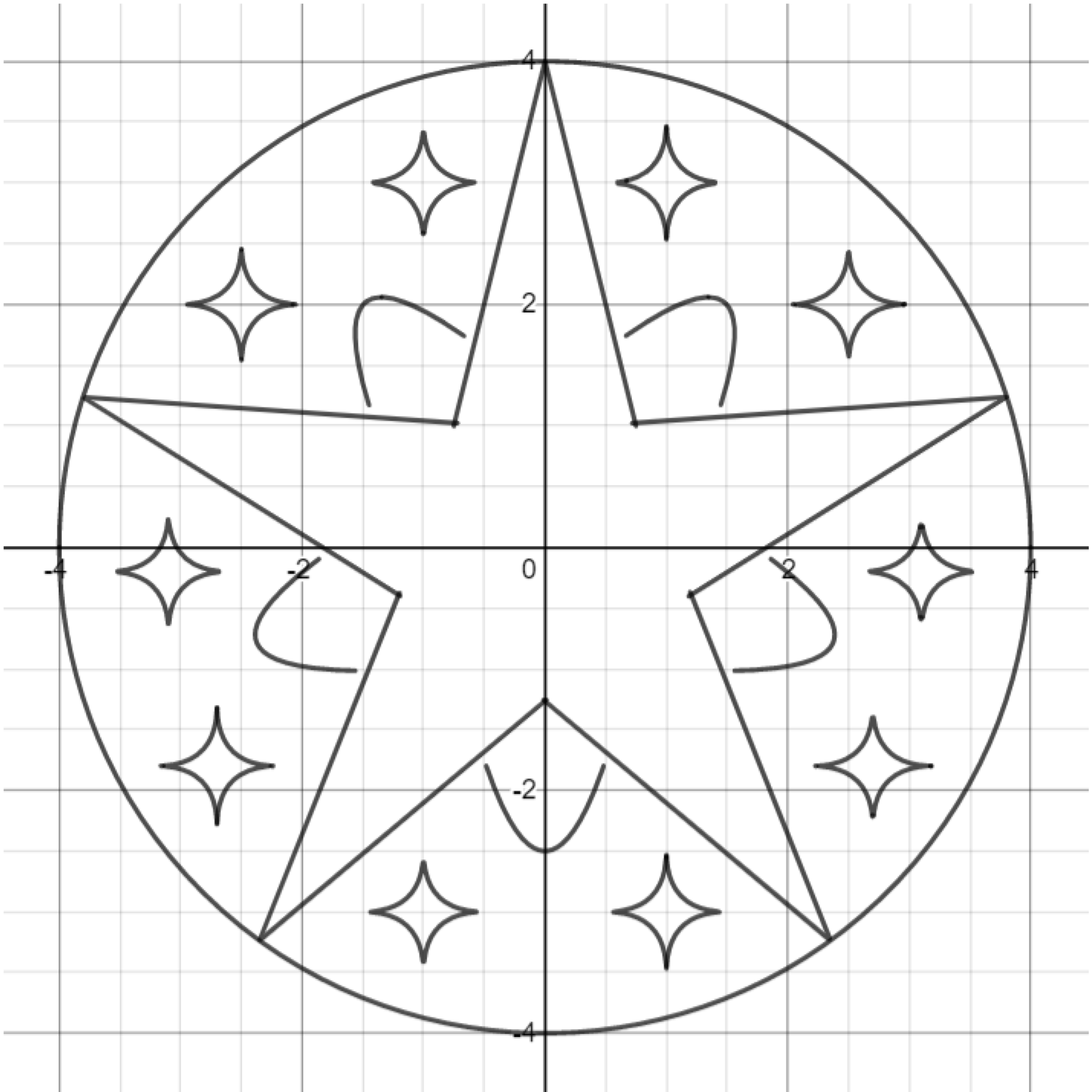# Graphing Calculator Drawing: Rotation

This post is part of a series.

Demonstration - Rotation. Observe the graph as you type each of the following inputs. In general, a graph can be rotated by an angle of $\theta$ about the origin by replacing $x$ and $y$ with the following expressions:

\begin{align*} x &\rightarrow x\cos\theta + y\sin\theta \\[5pt] y &\rightarrow y\cos\theta - x\sin\theta \end{align*}

Note that $\theta$ should be given in radians, and one can convert degrees to radians by multiplying by the conversion factor $\frac{\pi}{180}.$

\begin{align*} &y\cos \frac{\pi}{6} - x \sin \frac{\pi}{6} = \left( x \cos \frac{\pi}{6} + y\sin\frac{\pi}{6} \right)^2 \\[10pt] &\left( \frac{x \cos \frac{\pi}{4} + y \sin \frac{\pi}{4} }{4} \right)^{2}+\left( \frac{ y \cos \frac{\pi}{4} - x \sin \frac{\pi}{4} }{2} \right)^{2}=1 \end{align*}

Exercise. Reproduce the graph below by drawing an absolute value function and then rotating it a fifth of a circle counterclockwise.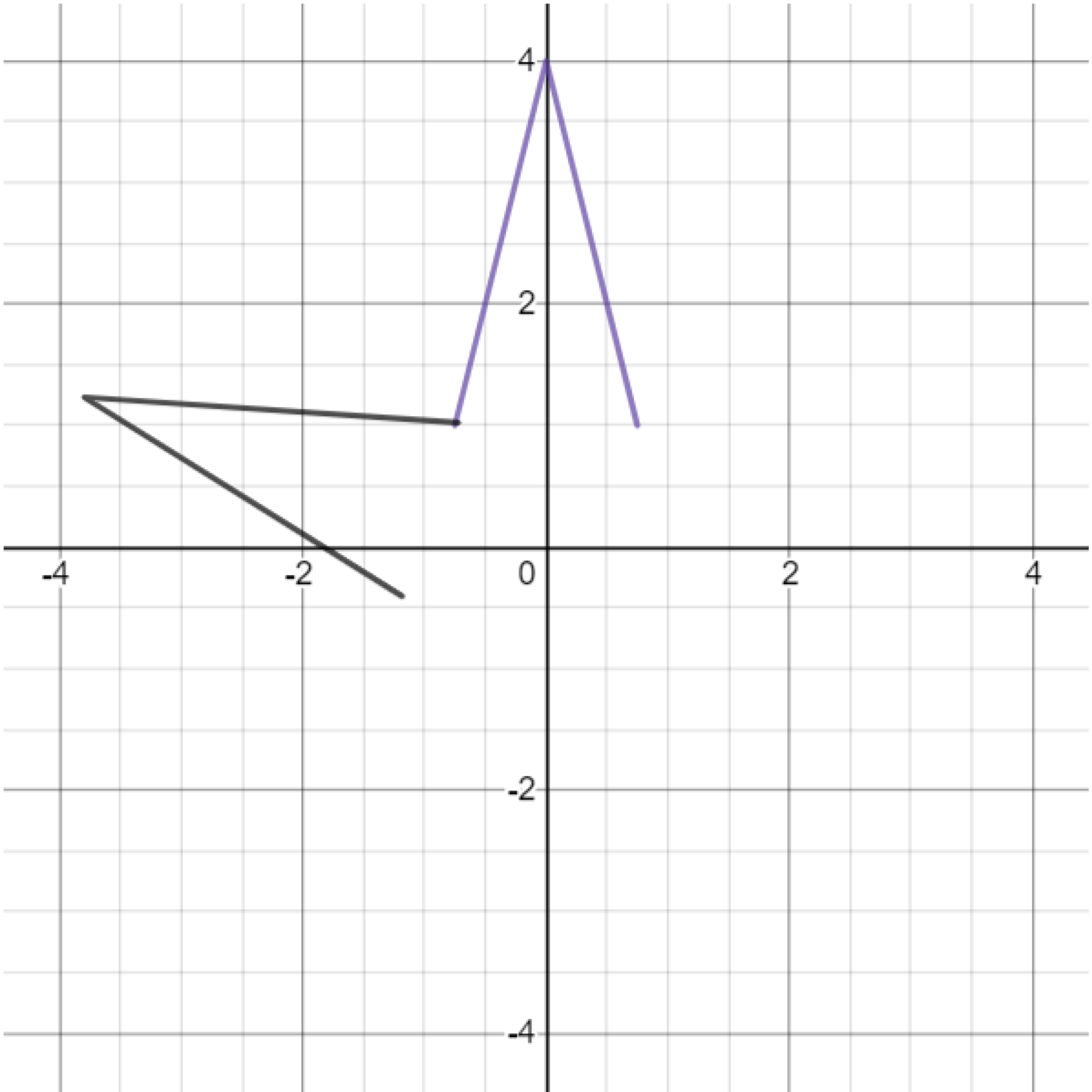Exercise. Continue drawing rotated absolute value functions to form a star.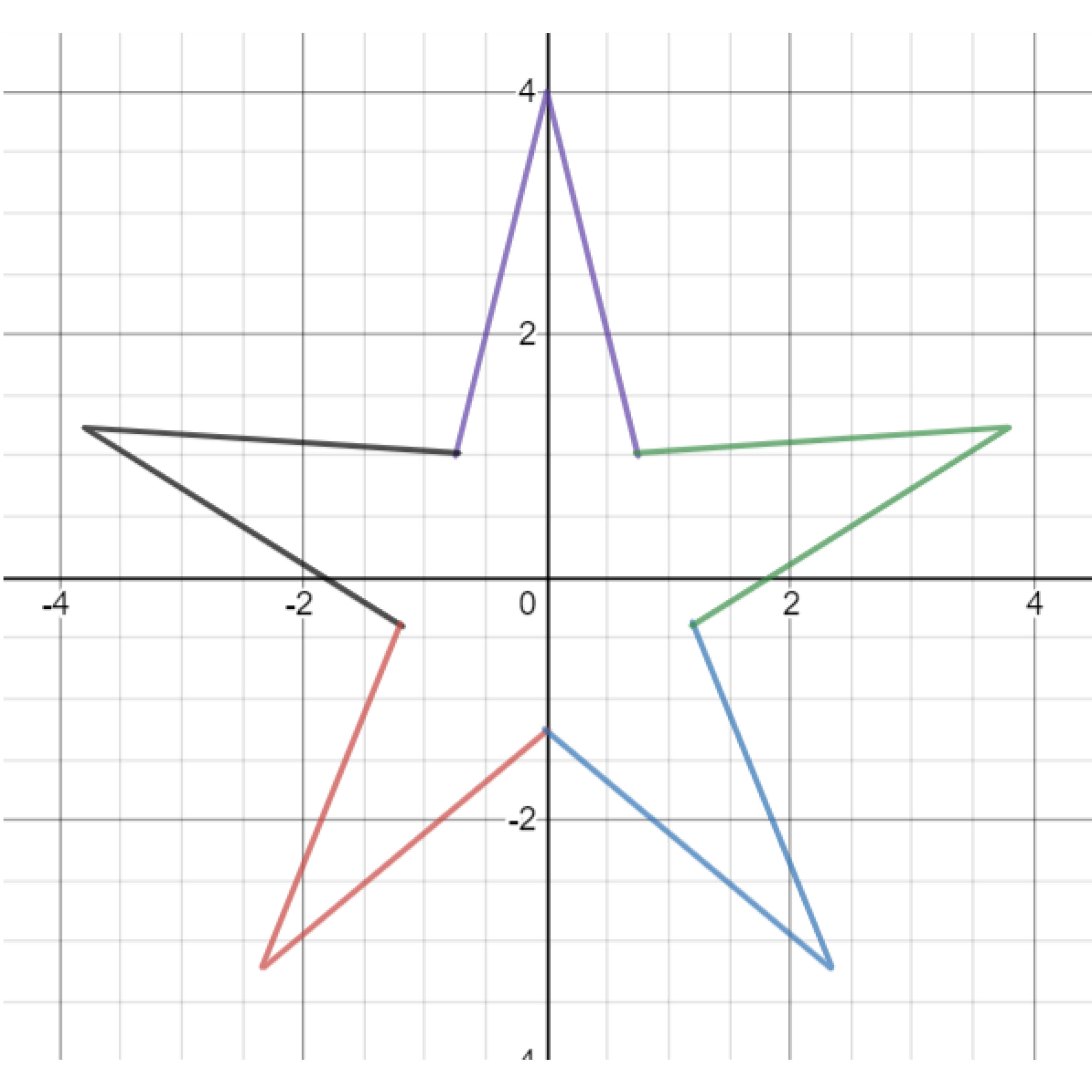Exercise. Draw a circle that passes through the sharp points of the star.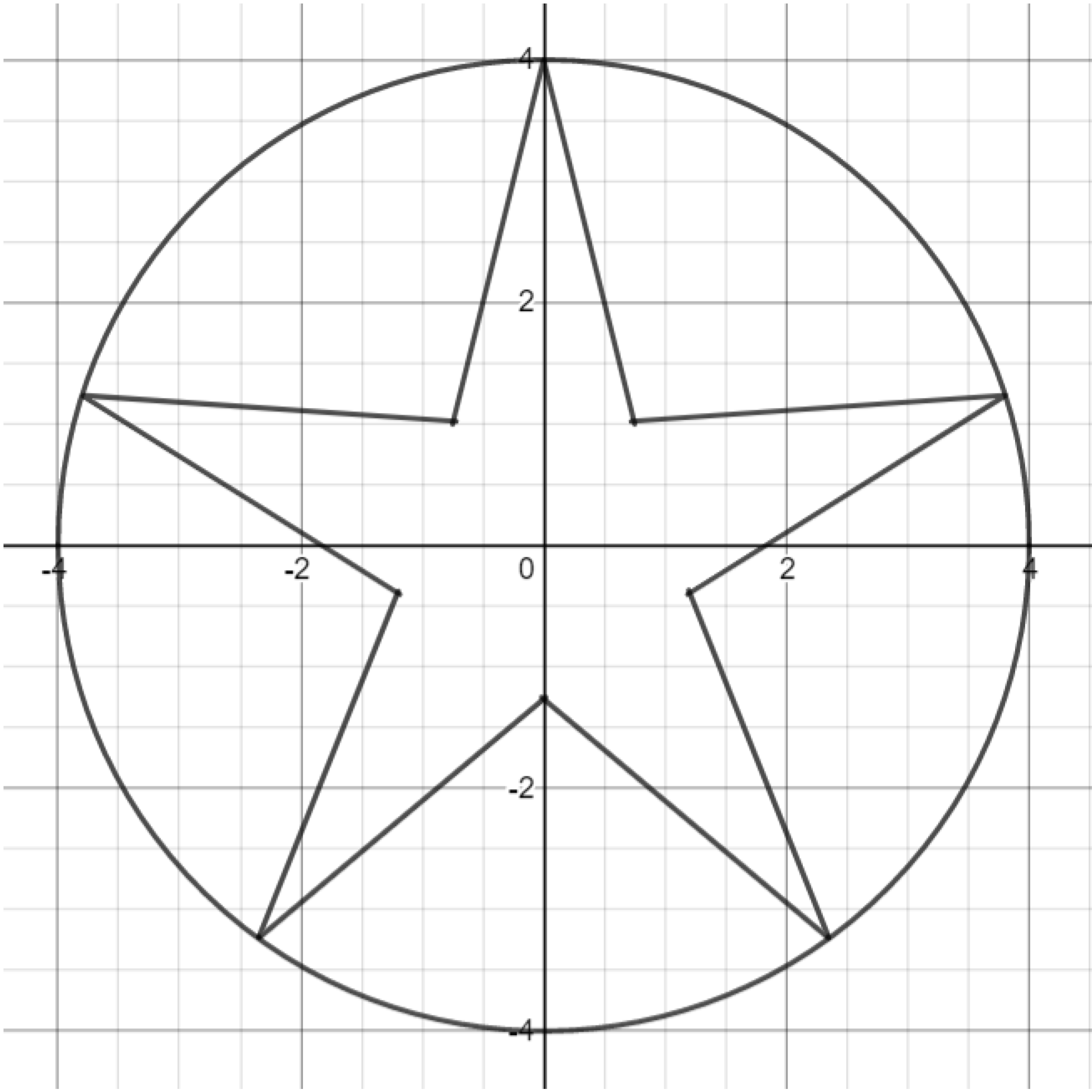Exercise. Add a background layer by drawing rotated parabolas.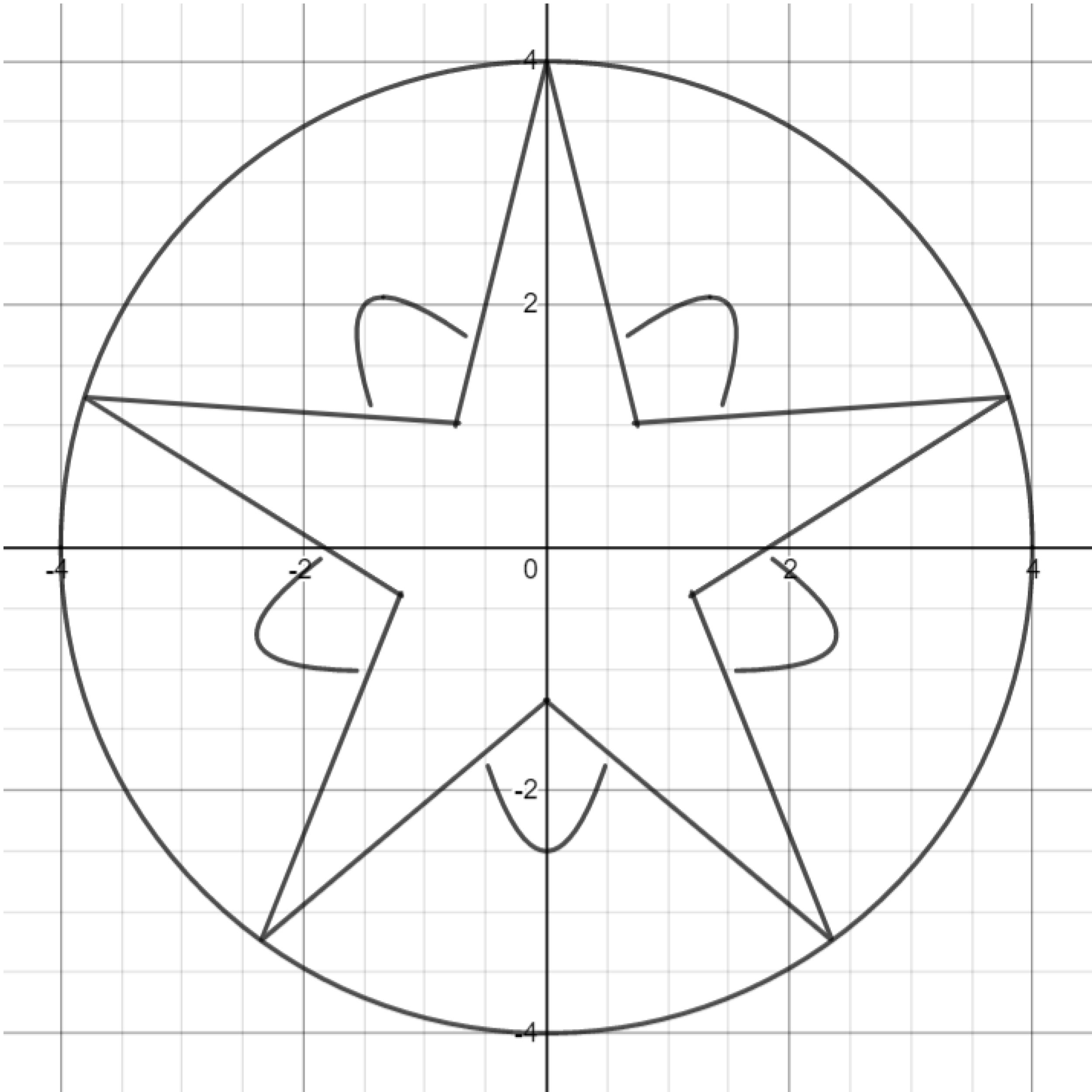Exercise. Finally, add non-Euclidean ellipses to the background.